GeeksforGeeks App
Open AppBrowser
Continue

## Related Articles

Ohm’s Law provides a relationship between current, resistance, and voltage across an electrical circuit. This relationship between current I, voltage V, and resistance R was given by famous German scientist Georg Simon Ohm in 1827. He found conducting his experiment that the product of the current flowing through the conductor and the resistance of the conductor determines the voltage drop over that conductor in the circuit.

In this article, we’ll learn about What is Ohm’s Law?, Ohm’s Law Formula, its experimental verification, Ohm’s Law Magic triangle and pie chart, the determination of power using Ohm’s Law, Limitations and Applications of Ohm’s Law.

## What is Ohm’s Law?

Ohm’s law states that the voltage across a conductor is directly proportional to the current flowing through it, provided all physical conditions and temperatures remain constant.

Hence, according to Ohm’s Law, the current flowing through the conductor is directly proportional to the voltage across the circuit, i.e. V ∝ I. Thus, as Ohm’s Law provides the basic relation between the voltage applied and current through the conductor, it is considered as the basic law which helps us in dealing electric circuit.

Ohm’s Law states that the current follows a linear relationship with voltage.

## Ohm’s Law Formula

Under the condition that all physical parameters and temperatures remain constant, Ohm’s law states that the voltage across a conductor is directly proportional to the current flowing through it.

Ohm’s Law is stated as:

V ∝ I

V = I × R

where
R is the Constant of Proportionality known as Resistance
V is the Voltage applied
I is the current flowing through the electrical circuit.

The above formula can be rearranged to calculate current and resistance also, as follows:

According to Ohm’s Law, the current flowing through the conductor is,

I = V / R

Similarly, resistance can be defined as,

R = V / I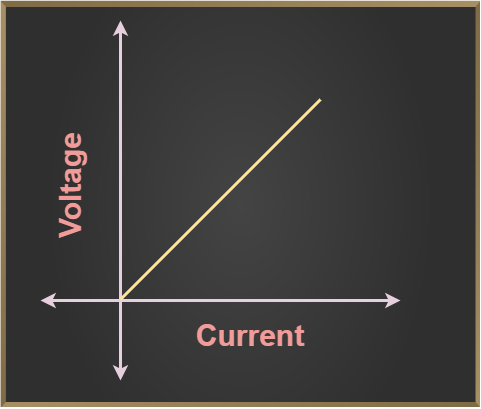Ohm’s Law holds good when physical conditions provided like temperature are constant. This is because of the fact that the current flowing through the circuit varies by changing the temperature. Therefore, in such cases when physical factors like temperature come into play, Ohm’s law violates. For example, in the case of a Light bulb, where temperature increases when the current flowing through it rises. Here, Ohm’s Law doesn’t follow.

## Ohm’s Law Formulas

Ohm’s law provides three formulas or equations which are:

V = I × R

I = V / R

R = V / I

where,
V is the voltage
I is the resistance
R is the resistance

## Ohm’s Law Triangle

Ohm’s Law Triangle is a visual representation for understanding and learning the Ohm’s Law relation between voltage, current, and resistance. This tool help helps engineers to remember the order of the relationship between the three main aspects: current (I), voltage (V), and resistance (R).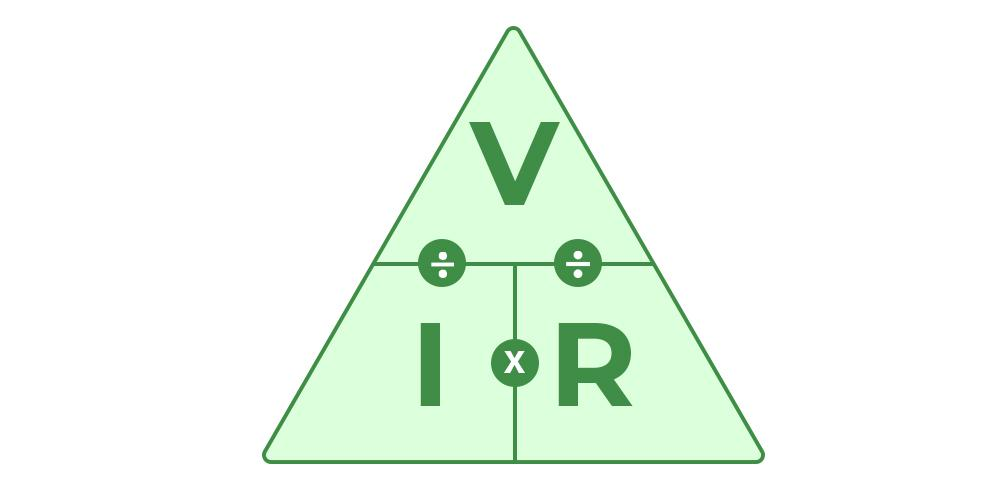## Vector Form of Ohm’s Law

The relation between current and voltage is established by, Ohm’s law and its vector form is,where,is Current Density vector,is Electric Field vector,
σ is conductivity of material.

### Resistivity

The hindrance faced by the electrons while moving in any material is called the resistivity of the material.

Let a resistor of a length of ‘l’ and the cross-sectional area of ‘A’ has a resistance be R.

Then we know,

Resistance is directly proportional to the length of the resistor, i.e. R ∝ l, and…(1)

Resistance is inversely proportional to the cross-section area of the resistor, i.e. R ∝ 1/A…(2)

combining eq. (1) and eq.(2)

R = ρl / A

where
ρ is the proportionality constant called coefficient of resistance or resistivity

Now if L = 1m and A = 1m2, in the above formula we get,

R = ρ

which means for a resistor of length 1 m and cross-section area 1 m2 the resistance is called the resistivity of the material.

## Experimental Verification of Ohm’s Law

Verification of Ohm’s Law is achieved by performing the following experiment.

### Apparatus Required

The apparatus required for performing the experiment for the Verification of Ohm’s Law is,

• Resistor
• Ammeter
• Voltmeter
• Battery
• Plug Key
• Rheostat

### Circuit Diagram

The circuit diagram for the Experimental Verification of Ohm’s Law is given in the diagram below,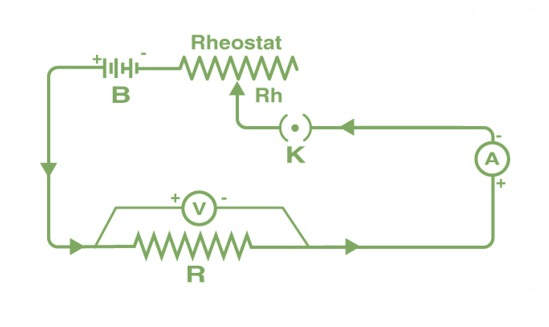### Procedure

• The key K is closed initially and the rheostat is adjusted such that the reading in ammeter A and voltmeter V is minimum.
• The current is then increased in the circuit by adjusting the rheostat, and the current at various values of the rheostat and their respective voltage is recorded.
• Now for different values of voltage(V) and current(I) and then calculate the ratio of V/I.
• After calculating all the ratios of V/I for different values of voltage and current, we notice that the value is almost constant.
• Now plotting a graph of the current against the potential difference we get a straight line. This shows that the current is directly proportional to the potential difference and its slope is the resistance of the wire.

## Ohm’s Law Pie Chart

To better understand the relationship between various parameters, we can take all the equations used to find the voltage, current, resistance, and power, and condense them into a simple Ohm’s Law pie chart as shown below.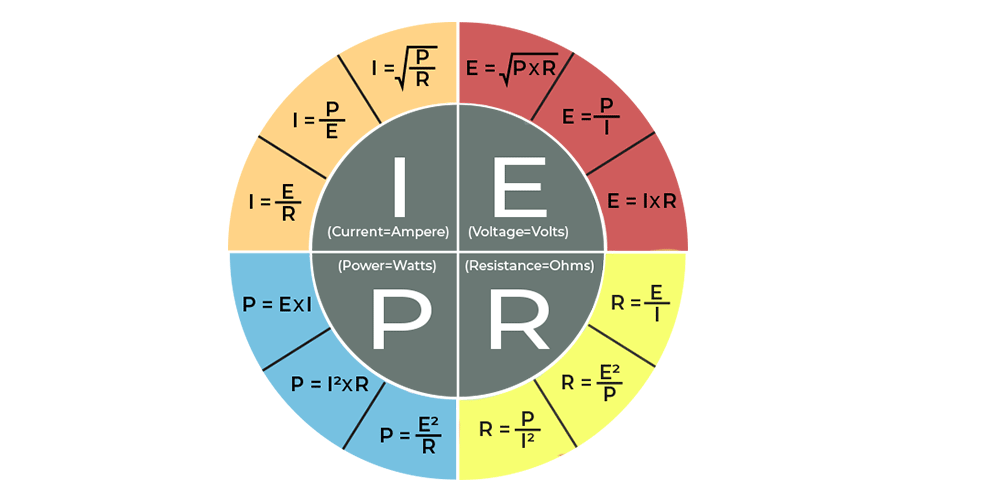## Ohm’s Law Matrix Table

Like Ohm’s Law Pie Chart shown above, we can condense the individual Ohm’s Law equations into a simple matrix table as shown below for easy reference when calculating an unknown value.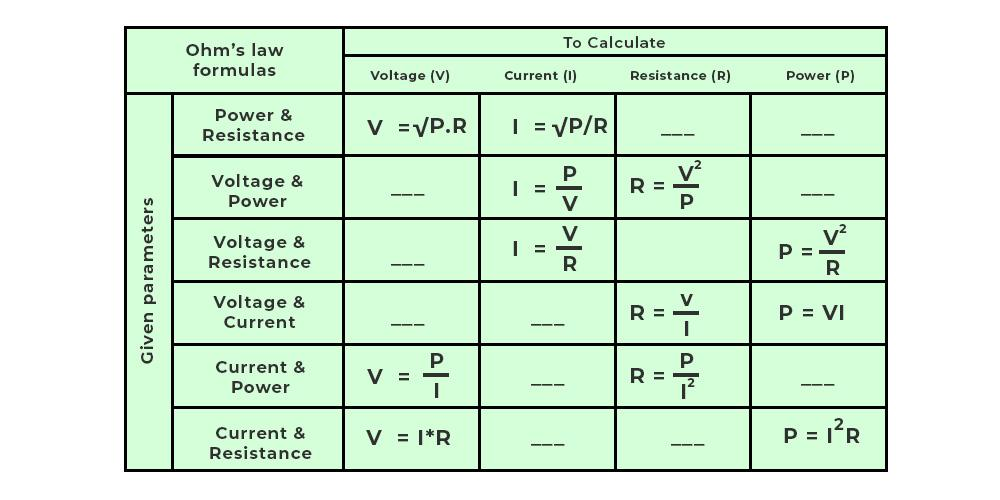## Applications of Ohm’s Law

When the other two numbers are known, Ohm’s law may be used to determine the voltage, current, impedance, or resistance of a linear electric circuit.

Main applications of Ohm’s Law:

• It also simplifies power calculations.
• To keep the desired voltage drop between the electrical components, Ohm’s law is employed.
• An electric circuit’s voltage, resistance, or current must be determined.
• Ohm’s law is also utilized to redirect current in DC ammeters and other DC shunts.

## How do we Establish Current-Voltage Relationship?

The ratio V ⁄ I remains constant for a given resistance while establishing the current-voltage connection, hence a graph of the potential difference (V) and current (I) must be a straight line.

How can we discover the unknown resistance values?

The constant ratio is what determines the unknown resistance values. The resistance of a wire with a uniform cross-section relies on the length (L) and the cross-section area (A). It also relies on the conductor’s temperature.

The resistance, at a given temperature,

R = ρ L ⁄ A

Here, ρ is the specific resistance or resistivity and is the wire material’s characteristic.

The wire material’s specific resistance or resistivity is,

ρ = R A ⁄ L

## Limitations of Ohms Law

• The law of Ohm does not apply to unilateral networks. The current can only flow in one direction in unilateral networks. Diodes, transistors, and other electronic components are used in these sorts of networks.
• Non-linear components are also exempt from Ohm’s law. Non-linear components have a current that is not proportional to the applied voltage, which implies that the resistance value of those elements varies depending on the voltage and current. The thyristor is an example of a non-linear element.

## Analogies of Ohm’s Law

Various analogies of Ohm’s Law are,

### Hydraulic Analogy

A hydraulic circuit can be compared to an ohmic conductor to make the problem easier to solve. Here, pressure works similarly to voltage, and flow rate works similarly to current.

### Temperature Analogy

Similarly, a temperature circuit can also be compared to an ohmic conductor. Here, temperature gradient works similarly to voltage, and heat flow works similarly to current.

## Solved Examples on Ohm’s Law

Example 1: Find the resistance of an electrical circuit with a voltage supply of 15 V and a current of 3 mA.

Solution:

Given:

V = 15 V,

I = 3 mA = 0.003 A

The resistance of an electrical circuit is given as:

R = V / I

= 15 V / 0.003 A
= 5000 Ω
= 5 kΩ

Hence, the resistance of an electrical circuit is 5 kΩ.

Example 2: If the resistance of an electric iron is 10 Ω and a current of 6 A flows through the resistance. Find the voltage between two points.

Solution:

Given:

I = 6 A, R = 10 Ω

The formula to calculate the voltage is given as:

V = I × R

V = 6 A × 10 Ω
= 60 V

Hence, the voltage between two points is 60 V.

Example 3: Find the current passing through the conductor drawing 20 volts when the power drawn by it is 60 watts.

Solution:

According to Ohm’s P = VI

Given P = 60 watt, V = 20 volt

I = P/V
= 60/20
= 3 A

Hence, the current flowing through the conductor is 3 A

## FAQs on Ohm’s Law

### Question 1: State the Ohm’s Law.

According to Ohm’s Law the current passing through the conductor is directly proportional to the potential difference across the end on the conductor, if temperature and the other physical conditions doesn’t changes.

### Question 2: What is the unit of resistance?

The SI unit of resistance is Ohm. It is denoted by  Ω.

### Question 3: What is the dimensional formula for resistance?

Dimensional formula for resistance is [M1L2T-3I-2]

### Question 4: Why doesn’t Ohm’s law apply to semiconductors?

Semiconducting devices are nonlinear in nature due to which Ohm’s law does not apply to them. This indicates that the voltage-to-current ratio does not remain constant when voltage varies.